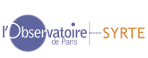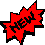BACK
HOME SHOW THIS PAGE
ATMOSPHERIC AND OCEANIC EXCITATION OF THE NUTATION
 ATMOSPHERIC AND OCEANIC EXCITATION OF THE NUTATION (1984 to nowadays) updated : May 2010The following tool allows you to study the atmospheric and oceanic excitation of the nutation. Equatorial excitation functions associated with the nutation of the Earth are compared to the Celestial Atmospheric Angular Momentum (CEAM) and Celestial Oceanic Angular Momentum (COAM) functions through visual plot and computation of the correlation coefficients. Both quantities can be taken into separately or added up.

 Inverted barameter model         Non Inverted barameter model             Civil date for graphics Comparison of observed excitation with : Matter + motion terms    Matter term     Motion term Atmospheric excitation NCEP (1984-current year)        Oceanic excitation ECCO (1993-2009) First date : year month day     Last date : year month day Plot and correlate X comp. of χ'G and χ''A/O (along vernal point) Plot and correlate Y comp. of χ'G and χ''A/O (90į)   Parameters of observed celestial equatorial excitation functions (see explanations below):   Chandler period days   Chandler quality factor   FCN period days         FCN quality factor       ap days   aw Apply Vondrak frequency filter Remove parabolic trend Select band above Select band below Select band around Remove band around (P0) year    Transfer coefficient for P0 :T0= % The Vondrak filter transfer function at another period P is given by : T=1/(1+(P0/P)6 (1-T0)/T0) For the case "Select band below" the selected period is transfered with a rate of (100-T0) Remove circular periodic components (periods in year) in both functions χ'G and χ''Fand polynomial of degree before comparison Produce data file χ'G /χ'A/O χ''A/O (unit : milliarcseconds ; no filtering, no fit)
 The observed (or geodetic) celestial equatorial excitation functions are computed from the IERS C04 series (sampling of 1 day, fluctuations > 6 days). Let P=dX + idY be the Celestial Pole Offsets, referred to nutation model UAI 2000, the complex form of those functions is :       χ'G = P + i ( 1/σ'FCN+ 1/σ'C) dP/dt - 1/(σ'C σ'FCN)d2P/dt2 where σ'FCN = 2π / TFCN (1 + i/2QFCN) ; σ'C = Ω + 2π / TC (1 + i/2QC) with Ω the Earth angular velocity The celestial equatorial excitation functions are based upon the knowledge of the Chandler term period TC and its quality factor QC, the Free Core Nutation (FCN) period TFCN and its quality factor QFCN. As these parameters are affected by large uncertainties, we let you the possibility to tune them within the allowed bands (426